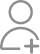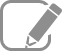Take Class 9 Tuition from the Best Tutors

•Affordable fees
•1-1 or Group class
•Flexible Timings
•Verified Tutors

Search in

# An object of mass 40 kg is raised to a height of 5 m above the ground. What is its potential energy? If the object is allowed to fall, find its kinetic energy when it is half-way down.

Learn NCERT Exercise 11Follow 1AnswerGravitational potential energy is given by the expression, W = mgh Where, h = Vertical displacement = 5 m m = Mass of the object = 40 kg g = Acceleration due to gravity = 9.8 m s−2 ∴ W = 40 × 5 × 9.8 = 1960 J. At half-way down, the potential energy of the object will be = 980...

Gravitational potential energy is given by the expression,

W = mgh

Where,

h = Vertical displacement = 5 m

m = Mass of the object = 40 kg

g = Acceleration due to gravity = 9.8 m s−2

∴ W = 40 × 5 × 9.8 = 1960 J.

At half-way down, the potential energy of the object will be= 980 J.

At this point, the object has an equal amount of potential and kinetic energy. This is due to the law of conservation of energy. Hence, half-way down, the kinetic energy of the object will be 980 J.

Experienced and certified Tutor for classes till 10th

Mass (m) = 40 kgAcceleration due to gravity (g)= 10m/s²Height (h)= 5m Potential energy= m × g× hP.E= 40 × 10 × 5 = 2000J Potential energy = 2000J ( 2000 joules) At a height of 5 metre object has a potential energy of 2000 J.When this object is allowed to fall and it is...

Mass (m) = 40 kg
Acceleration due to gravity (g)= 10m/s²
Height (h)= 5m

Potential energy= m × g× h
P.E= 40 × 10 × 5 = 2000J

Potential energy = 2000J ( 2000 joules)

At a height of 5 metre object has a potential energy of 2000 J.
When this object is allowed to fall and it is Half way down its height above the ground will be half of 5 m= 5/2= 2.5m.

P.E at Half way down= m× g×h
P.E= 40× 10 × 2.5= 1000J
[h= 2.5 m]

Potential Energy at Half way down= 1000 joules.

According to law of conservation of energy:

Total potential energy= potential energy at Half way down+ kinetic energy at Half way of a down

2000 = 1000 + K.E at Half way down

K.E at Half way down= 2000- 1000= 1000J

Kinetic energy at half way down= 1000 joules.

Now ask question in any of the 1000+ Categories, and get Answers from Tutors and Trainers on UrbanPro.com

Find Class 9 Tuition near you

Looking for Class 9 Tuition ?

Learn from the Best Tutors on UrbanPro

Are you a Tutor or Training Institute?

Join UrbanPro Today to find students near you
X

### Looking for Class 9 Tuition Classes?

The best tutors for Class 9 Tuition Classes are on UrbanPro

• Select the best Tutor
• Book & Attend a Free Demo
• Pay and start Learning### Take Class 9 Tuition with the Best Tutors

The best Tutors for Class 9 Tuition Classes are on UrbanPro